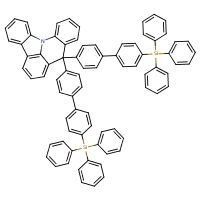Structure InformationCompound Identification

SMILES

C1=CC=C(C=C1)[Si](C1=CC=CC=C1)(C1=CC=CC=C1)C1=CC=C(C=C1)C1=CC=C(C=C1)C1(C2=CC=C(C=C2)C2=CC=C(C=C2)[Si](C2=CC=CC=C2)(C2=CC=CC=C2)C2=CC=CC=C2)C2=CC=CC3=C2N(C2=CC=CC=C32)C2=CC=CC=C12

InChIKey

InChIKey=QCFOIBJRRDFIGD-UHFFFAOYSA-N

Formula

C79H57NSi2

Mass

1076.502

### Export to:

Taxonomic Classification

Taxonomy Tree

Kingdom

Organic compounds

Superclass

Organoheterocyclic compounds

Class

Quinolines and derivatives

Subclass

Benzoquinolines

Intermediate Tree Nodes

Acridines

Direct Parent

Pyrroloacridines

Alternative Parents

Molecular Framework

Aromatic heteropolycyclic compounds

Substituents

Phenylquinoline - Pyrroloacridine - Carbazole - Biphenyl - Indole - Indole or derivatives - Monocyclic benzene moiety - Benzenoid - Heteroaromatic compound - Pyrrole - Organic metalloid salt - Azacycle - Organonitrogen compound - Organic metalloid moeity - Hydrocarbon derivative - Organosilicon compound - Organic nitrogen compound - Aromatic heteropolycyclic compound

Description

This compound belongs to the class of organic compounds known as pyrroloacridines. These are compounds containing a pyrroloacridine moiety, which is a tetracyclic heterocycle which consists of a pyrrole ring fused to an acridine.

External Descriptors

Not available